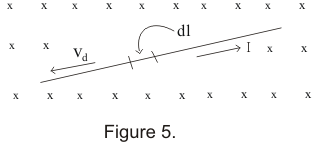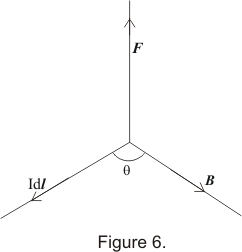Magnetic force on a current carrying wire

(6) Magnetic force on a current carrying wire

• We know that current flowing in a conductor is nothing but the drift of free electron's from lower potential end of the conductor to the higher potential end
• when a current carrying conductor is placed in a magnetic field ,magnetic forces are exerted on the moving charges with in the conductor
• Equation -1 which gives force on a moving charge in a magnetic field can also be used for calculating the magnetic force exerted by magnetic field on a current carrying conductor (or wire)
• Let us consider a straight conducting wire carrying current I is placed in a magnetic field B(x).Consider a small element dl of the qire as shown below in the figure• Drift velocity of electrons in a conductor and current I flowing in the conductor is given by I=neAvd
Where A is the area of cross-section of the wire and n is the number of free electrons per unit volume
• Magnetic force experienced by each electron in presence of magnetic field is
F=e(vd X B)
where e is the amount of charge on an electron
• Total number of electron in length dl of the wire
• Thus magnetic force on wire of length dl is
if we denote length dl along the direction of current by the vector dl the above equation becomes
dF=(nAevd)(dl X B)
or dF=I(dl X B)                   -- (12)
where the quantity IdL is known as current element
• If a straight wire of length l carrying current I is placed in a uniform magnetic field then force on wire would be equal to
dF=I(L X B)                   -- (13)

Direction of force
• Direction of force is always perpendicular to the plane containing the current element IdL and magnetic field B• Direction of force when current element IdL and B are perpendicular to each other can also be find using either of the following rules

i) Fleming'e left hand rule:-
If fore finger ,the middle finger and thumb of the left hand are stretched in such a way that the all are mutually perpendicular to each other then,if the fore finger points in the direction of the field (B) and middle finger points in the direction of current I ,the thumb will point in the direction of the force
ii) Right hand palm Rule:
Stretch the finger and thumb of the right hand so that they are perpendicular to each other .If the fingers point in the direction of current I and the palm in the direction of field B then the thumb will point in the direction of force

Note to our visitors :-

Thanks for visiting our website.
DISCLOSURE: THIS PAGE MAY CONTAIN AFFILIATE LINKS, MEANING I GET A COMMISSION IF YOU DECIDE TO MAKE A PURCHASE THROUGH MY LINKS, AT NO COST TO YOU. PLEASE READ MY DISCLOSURE FOR MORE INFO.